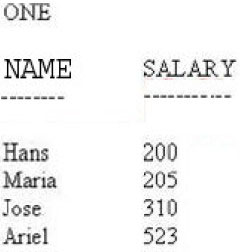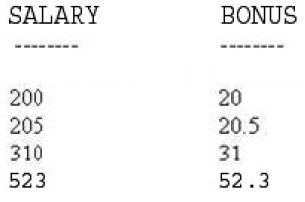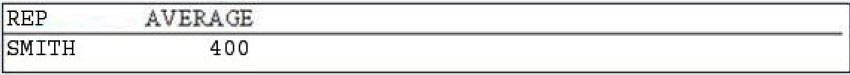# SAS Advanced Programming for SAS 9 v1.0

Page:    1 / 13
Exam contains 191 questions

The following SAS program is submitted:
data temp;
set sasuser.history(kep=date);
format date qtr
<insert BY statement here>
if first.date then total=0;
total+1;
if last.date;
run;
proc print data=temp;
run
SASUSER.HISTORY is sorted by the SAS date variable DATE.
The following output is required:

Date Total -
1 13
3 15
4 25
Which By statement completes the data step and successfully generates the required output?

• A. by groupformat date;
• B. by formateed date;
• C. by notsorted date;
• D. by date qtr

Which statement(s) in the DATASETS procedure alter(s) the name of a SAS data set stored in a SAS data library?

• A. MODIFY and CHANGE statements
• B. RENAME statement only
• C. CHANGE statement only
• D. MODIFY and RENAME statements

Given has SAS dataset ONE:The following SAS program is submitted:Proc sql;
<insert SQL clause here>
from one;
quit;
The following output is desired:
Which SQL procedure clause completes the program and generates the desired output?

• A. Select salary, salary*.10 var=BONUS
• B. Select salary, salary*.10 label='BONUS'
• C. Select salary, salary *.10 column='BONUS'
• D. Select salary, salary*.10 name='BONUS'

Given the non-indexed SAS data set TEMP:

TEMP -

X Y -
- -

P 52 -

P 45 -

A 13 -

A 56 -

R 34 -

R 12 -

R 78 -
The following SAS program is submitted:
Proc print data=temp;
<insert BY statement here>
run;
Which BY statement completes the program, creates a listing report that is grouped by X and completes without errors?

• A. By X notsorted;
• B. By X grouped;
• C. By Descending X;
• D. By X;

Given the data set SASHELP.CLASS

SASHELP.CLASS -

NAME AGE -

Mary 15 -

Philip 16 -

Robert 12 -

Ronald 15 -
The following SAS program is submitted
%let value = Philip;
proc print data =sashelp.class;
<insert Where statement here>
run;
Which WHERE statement successfully completes the program and produces a report?

• A. Where upcase(name)="upcase(&value)";
• B. Where upcase(name)="%upcase(&value)";
• C. Where upcase(name)=upcase(&value);
• D. Where upcase(name)=%upcase(&value);

Following SAS program is submitted:
data temp(<insert option here>);
infile 'rawdata';
input x \$ y z;
run;
RAWDATA is a file reference to an external file that is ordered by the variable X.
Which option specifies how the data in the SAS data set TEMP will be sorted?

• A. ORDEREDBY=X
• B. GROUPBY=X
• C. SORTEDBY=X
• D. SORTSYNC=X

Given the following partial SAS log:
NOTE: SQL table SASHELP.CLASS was created line
Create table SASHELP.CLASS(bufsize=4096)
(
Name char(8);
Gender Char(1);
Age num;
Height num;

Weight num -
);
Which SQL procedure statement generated this output?

• A. DESCRIBE TABLE
• B. LIST TABLE
• C. VALIDATE TABLE
• D. CREATE TABLE

The following SAS program is submitted:
options mprint;
%macro test(parm);
proc &parm data = sashelp.prdsale;
run;
%mend;
%test(print)
What is the result of the MPRINT options?

• A. It has no effect in this example
• B. It writes the original program code inside the marco definition to the SAS log
• C. It writes macro execution messages to the SAS.log
• D. It echoes the text sent to the SAS compiler as a result of macro execution in the SAS log

Given the SAS data set ONE:

ONE -

REP COST -

SMITH 200 -

SMITH 400 -

JONES 100 -

SMITH 600 -

JONES 100 -
The following SAS program is submitted:
Proc sql;
Select rep, avg(cost) as AVERAGE

From one -

Group by rep -
<insert SQL procedure clause here>
quit;
The following output is desired:Which SQL procedure clause completes the program and generates the desired output?

• A. having avg(cost) < select avg(cost) from one);
• B. Having avg(cost)>(select avg(cost) from one);
• C. Where avg(cost)>(select avg(cost) from one);
• D. Where calculated average > (select avg(cost) from one);

Given the data set SASHELP.CLASS:

SASHELP.CLASS -

NAME AGE -

Mary 15 -

Philip 16 -

Robert 12 -

Ronald 15 -
The following SAS program is submitted:
%let value = Philip;
proc print data = sashelp.class;
<insert WHERE statement here>
run;
Which WHERE statement successfully completes the program and procedures a report?

• A. Where upcase(name)=%upcase(&value);
• B. Where upcase(name)="upcase(&value)";
• C. Where upcase(name)=upcase(&value);
• D. Where upcase(name)="%upcase(&value)";

Given the SAS dataset ONE -

ONE -

SALARY -
200
205
523
The following SAS program is submitted
Proc sql;

Select * from one -
<Insert Where expression here>;
quit;
The following output is desired:

SALARY -
200
205
523
Which WHERE expression completes the program and generates the desired output?

• A. Where salary is not
• B. Where salary ne null
• C. Where salary is not missing
• D. Where salary ne missing

At the start of a new SAS session; the following program is submitted:
%macro one;
data _null_;
call symput('proc','measn);
run;
proc &proc data=sashelp.class;
run;
%mend;
%one()
What is the result?

• A. The marco variable PRCO is stored in the SAS catalog WORK.SASMACR
• B. The program fails to execute because PROC is a reserved word
• C. The macro variable PROC is stored in the local symbol table
• D. The macro variable PROC is stored in the global symbol table

The following SAS program is submitted:
%let value=9;
What is the value of the macro variable NEWVAL?

• A. null
• B. 2
• C. 1
• D. 1.8

Given the non-indexed SAS data set TEMP:

TEMP -

X Y -

P 52 -

P 45 -

A 13 -

A 56 -

R 34 -

R 12 -

R 78 -
The following SAS program is submitted:
Proc print data=temp;
<insert By statement here?
Run;
Which by statement completes the program, create a listing report that is grouped by X and completes without errors?

• A. X;
• B. By X groupd;
• C. By X notsorted;
• D. By descending X;

Which of the following is true about the COMPRESS=YES data set option?

• A. It is most effective with numeric data that represents large numeric values
• B. It is most effective with character data that contains patterns, rather than simple repetitions
• C. It uses the Ross Data Compression method to compress numeric data
• D. It is most effective with character data that contains repeated characters